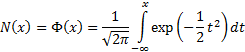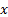/

### Enterprise Risk Management Formula Book Appendix A.7(a): Probability Distributions: Standard (i.e. unit) normal distribution: Cumulative distribution function

The table below tabulates the cumulative distribution function of the unit normal distribution, i.e.-       for values offrom 0.00 to 1.00 see here

-       for values offrom 1.00 to 2.00 see here

-       for values offrom 2.00 to 3.00 see here

-       for values offrom 3.00 to 4.00 see here